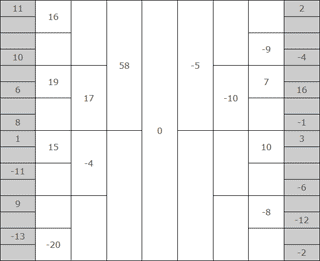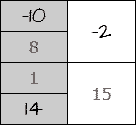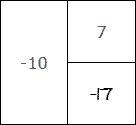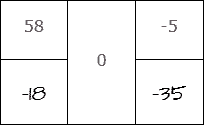# Welcome to Balance Quest!Balance Quest puzzles are mathemetical logic problems. Each puzzle contains a large grid comprised of grey and white cells - some are pre-filled with numbers, others are empty. Your task is to fill every empty cell with a positive or negative integer in such a way so that each white cell's value equals the sum of its adjoining half-height cells. When complete, each Balance Quest puzzle grid will "balance" itself in such a way so that the four center cells surrouding the center "zero cell" will always add up to zero. Each puzzle has one, and only one, unique solution.

There are four rules that must be followed in every Balance Quest puzzle:

• The grey cells must include all integers between -16 and 16, except zero.
• No number can be repeated within any of the grey cells.
• No number can be repeated within any of the white cells.
• The number in each white cell must equal the sum of its adjoining half-height cells.

# How the Grid Works

Each white square's value must always equal the sum of the values of the half-height squares immediately adjacent to it. Here are some examples of how this works:-10 + 8 = -2and1 + 14 = 15 -17 + 7 = -10 58 + -18 + -5 + -35 = 0 The center "zero cell" is always the sum of all four of its adjacent cells.